Pre účinnosť

Pre účinnosť bŕzd osobného vozidla je predpísané, že automobil pohybujúci sa po vodorovnej vozovke rýchlosťou 40km. H musí zastaviť na dráhe 15,4 m. Aké veľké je pritom spomalenie automobilu?

Result

a =  4.008 m/s2

Solution:Leave us a comment of this math problem and its solution (i.e. if it is still somewhat unclear...):Be the first to comment!Next similar math problems:

1. Theorem proveWe want to prove the sentence: If the natural number n is divisible by six, then n is divisible by three. From what assumption we started?
2. GravitationFrom the top of the 80m high tower, the body is thrown horizontally with an initial speed of 15 m/s. At what time and at what distance from the foot of the tower does the body hit the horizontal surface of the Earth? (use g = 10 ms-2)
3. Free fallThe free fall body has gone 10m in the last 0.5s. Find the body speed at the moment of impact.
4. G forcesCalculate deceleration of car (as multiple of gravitational acceleration g = 9.81 m/s2) which occurs when a car in a frontal collision slows down uniformly from a speed 111 km/h to 0 km/h in 1.2 meters trajectory.
5. Train 2The train slowed down from 90 km/h to 72 km/h in 5 seconds. How long track travel?
6. AccelerationThe car accelerates at rate 0.5m/s2. How long travels 400 meters and what will be its speed?
7. BrakesThe braking efficiency of a passenger car is required to stop at 12.5 m at an initial speed of 40 km/h. What is the acceleration braking by brakes?
8. DriverThe driver of the car at a speed of 100 km/h faced the obstacle and began to brake with a slowing of 5 m/s². What is the path to stopping the car when the driver has registered the obstacle with a delay of 0.7 s?
9. CollisionThe two bodies, whose initial distance is 240 m, move evenly against each other consistently. The first body has an initial velocity of 4 m/s and an acceleration of 3 m/s2, the second body has an initial speed of 6 m/s and an acceleration of 2 m/s2. Find
10. Against each other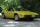From two points A, B distant 23 km at the same time started two cars against each other at speeds 41 km/h and 65 km/h. How long does cars meet and what distance passes each of them?
11. Znojmo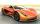From Znojmo to Brno started truck with a trailer at an average speed 53 km per hour. Against him 14 minutes later from Brno started car with an average speed of 1.2-times greater than the truck. How long and how far from Znojmo, they meet if the distanc
12. Rocket start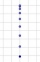The body launched vertically up returns to the start site in 6 seconds. What height did it have?
13. Average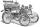What is the average speed of the car, where half of the distance covered passed at speed 66 km/h and the other half at 86 km/h.
14. BomberThe aircraft flies at an altitude of 4100 m above the ground at speed 777 km/h. At what horizontal distance from the point B should be release any body from the aircraft body to fall into point B? (g = 9.81 m/s2)
15. Reference angleFind the reference angle of each angle:
16. The positionThe position of a body at any time T is given by the displacement function S=t3-2t2-4t-8. Find its acceleration at each instant time when the velocity is zero.
17. Wind drift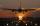The plane flies at 860 km/h, passing distance 3000 kilometers with the wind and once again against the wind for 6 h 59 min. What is the wind speed?